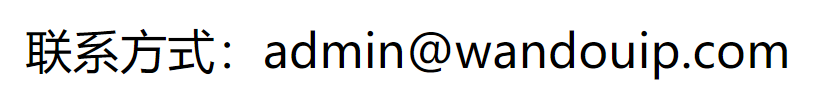## 源代码

``````class Solution {
public:
vector<int> multiply(const vector<int>& A) {

vector<int> B;
int res=1;
for(int i=0;i<A.size();i++)
{
for(int j=0;j<A.size();j++)
{
if(i!=j)
{
res=res*A[j];
}
}
B.push_back(res);
res=1;
}
return B;
}
};
``````15866163259

15866163259